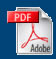Home English Maths Science ICT History Geography Art Year Six Revision Maths APP
Home > Mathematics > GCSE

The G.C.S.E. Maths SectionInformation about sampling and statisticsInformation about equation manipulationDrawing a graph of a cooling curvePractice Key Skills Application of NumberFour Operations with Negative NumbersBalancing Equations with NumbersEquation ManipulationSample Standard DeviationVolume of a pyramid.Problems with circlesFactorizing a Quadratic EquationReal Life Graphs

Past PapersHigher Specimen Unit 1 Higher Specimen Unit 2 Higher Specimen Unit 3 H Unit 1 Mark Scheme H Unit 2 Mark Scheme H Unit 3 Mark SchemeMar 13 Higher Unit 1 Mar 13 Higher Unit 2 Mar 13 Higher Unit 3 H Unit 1 Mark Scheme H Unit 2 Mark Scheme H Unit 3 Mark SchemeNov 12 Higher Unit 1 Nov 12 Higher Unit 2 Nov 12 Higher Unit 3 H Unit 1 Mark Scheme H Unit 2 Mark Scheme H Unit 3 Mark SchemeJun 12 Higher Unit 1 Jun 12 Higher Unit 2 Jun 12 Higher Unit 3 H Unit 1 Mark Scheme H Unit 2 Mark Scheme H Unit 3 Mark SchemeFoundation Unit 1 Specimen Foundation Unit 2 Specimen Foundation Unit 3 Specimen F Unit 1 Mark Scheme F Unit 2 Mark Scheme F Unit 3 Mark Scheme